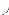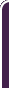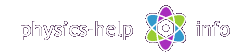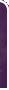homephysics online guidephysics help my info terms of use contactsWhat is Physics? Mechanics Electricity and Magnetism Electric Field Gauss' Law about below subjects Electric Potential Capacity Direct Current Magnetic Field Magnetic Field Laws Magnetic Interactions Electromagnetic Induction Maxwell's Equations Oscillations and Waves Simple Harmonic Motion Damped Harmonic Motion Driven Harmonic Motion Electric Oscillation Alternating Current Wave Motion Elastic Waves Electromagnetic Waves Optics Light Waves Geometrical Optics Interference Polarization Diffraction Fraunhofer Diffraction Dispersion, Absorption, Diffusion Doppler Effect Thermodynamics Ideal Gas Molecular Statistics Transport Phenomena First Law of Thermodynamics Second and Third Laws of Thermodynamics Imperfect Gas Liquids Solids Quantum Physics Thermal Radiation Quantum Properties of Light Wave Properties of Particles Planetary Model of Atom X-Rays Particle in Potential Well Pauli Exclusion Principle Nuclear Physics Solid State Physics Appendices# Electric Field   full version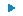SI units & Physics constants

Electricity is the branch of physics, which investigates interactions between electric chargesHere (all units see here):is electric force on charge q from Qis electric force on charge Q from qis position vector of charge q with respect to Qis electric field produced by charge Q near q

## General formulas

Point charge is a charge which dimensions are small with respect to distance between the charge and the point where the electric field from this charge is defined

The electric force vector between two point charges is defined by Coulomb’s lawwhereis permittivity of vacuumAnother form of Coulomb's lawwhereis Coulomb constant

The magnitude of electric forceor## Electric Charges

The electric charge of object is a scalar value given bywhere:

Np and Ne are numbers of protons and electrons in the object

## Charge Conservation Law

The Charge Conservation Law states that in any isolated system the net charge remains constantwhere n is total number of charges in the system

## The electric field

The electric field vector is defined byThe magnitude of electric fieldThe electric field produced by point charge Q at a distancefrom the chargeMagnitude of electric fieldHere P is a point where the electric field is defined

## Electric field lines

Any electric field can be defined graphically by means of the electric field lines, as shown belowThe electric field lines are drawn as curves so that the tangent line to the curve at arbitrary point P is directed along the vector of the electric field at this point, and the density of lines is directly proportional to the magnitude of the electric fieldwhere:

N is the number of lines crossing a small area A, oriented normally to the electric field with the center at the point P

s is scale coefficient, which is the same for all points

The electric field lines from point chargewhereis area of sphere with radius r  and center at the charge Q

## Uniform electric field

The uniform electric field is electric field where the vectoris constant everywhere in magnitude and direction## Superposition Principle

The Superposition Principle states that net electric field produced at any point by a system of charges is equal to the vector sum of all individual fields, produced by each charge at this pointwhere n is total number of charges in the system

The Superposition Principle for two point chargeswhereandare position vectors of point P with respect to the charges Q1 and Q2 respectivelyThe Superposition Principle for system of n point chargeswhereis position vector of point P with respect to the chargefor i = 1, 2, 3,..., n

The Superposition Principle for charge continuously distributed over an objectwhere:is radius position of point where the electric field is defined with respect to small volume dV with volume charge densityV is volume of the charged object

Electric field produced by uniformly charged ring at its axiswith magnitudewhere:is radius position of point P where the electric field is defined with respect to center of the ring

R is radius of ring

Q is total charge of the ringhome  |  physics online guide  |  physics help  |  my info  |  terms of use  |  contacts Dr. Nikitin © 2010